Skip to content
Related Articles
Data Handling
• Last Updated : 22 Mar, 2021

Nowadays, managing and representing data systematically has become very important especially when the data provided is large and complex, This is when Data Handling comes into the picture. The definition of Data handling is in the title itself, that is, Handling the data in such a way that it becomes easier for people to understand and comprehend the given information. Hence, The process of collecting, Recording, and representing data in some form of graph or chart to make it easy for people to understand is called Data handling.

Statistics is another term for data handling, and it is useful not only in the field of Math and Science but also in the fields where the representation of data is required. Let’s learn about some forms of Data handling, and how they work.

### Pictographs

A pictograph is the pictorial representation of any data given to us in written form. It can be said that pictograph used to be the earliest form of conversation, since way back in time, people have communicated mostly through pictures with each other since the languages were not present.

Indeed, Pictograph plays a role in our day-to-day life too. For instance, when a friend tells us a story, we start imagining the story in our head and that makes it both easy to understand and easy to remember for a long time for us.

### Drawing a Pictograph

Let’s learn to draw the pictograph with the help of an example,

Example: In a reading competition, there were three students participating- Rahul, Saumya, Ankush. They were supposed to read as many books as they can in an hour. Rahul read 3 books, Saumya read 2 books and Ankush read 4 books. Draw the pictograph for the information.

Solution:

There are some basic steps to draw a Pictograph:

• Decide the particular picture/pictures that is required to represent data, make sure that the picture is a little related in order to memorize information easily.
• Here, to successfully read a book, a smiley is denoted.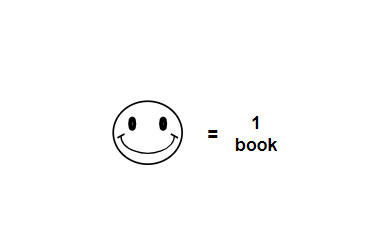• Now, draw the pictures according to information presented, for example, there will be 3 smilies for Rahul as he completed 3 books in an hour.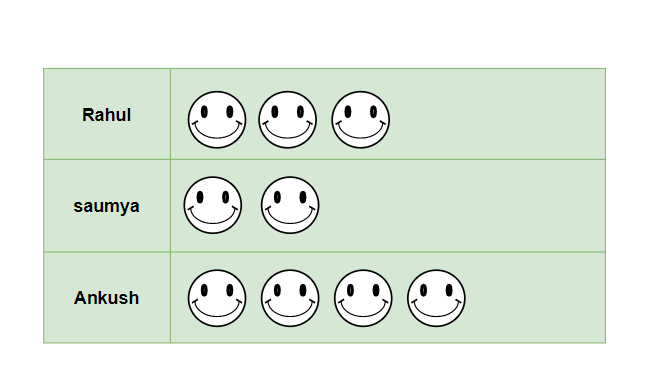### Sample Questions on Pictograph

Question 1: In a Theater, there are 3 Plays with different amounts of actors participating in each play. In play 1, there are 9 actors, in play 2, there are 3 lesser actors, and the number of actors in play 3 is one lesser than play 1. Draw the Pictograph for the information given and analyze in which play, the stage will be most crowded.

Solution:

From the information given in the question, we can say that play 1 has 9 actors, play 2 has 6 actors and play 3 has 10 actors

Representing actors in the pictorial form as,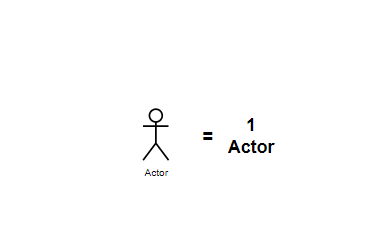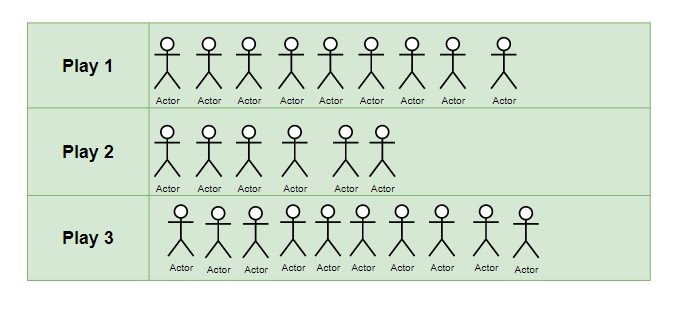Therefore, we can conclude that Play 3 has the stage most crowded as it has 10 actors acting on stage.

Question 2: In a Weather Report conducted for 5 weeks continuously, it was noted that not all days are sunny days in the season of spring. The observation said that week 1 had 4 sunny days, week 2 had 5 sunny days, week 3 had only 2 sunny days, week 4 had sunny days in the entire week, and week 5 had only 3 sunny days.

Draw a Pictograph for the information given above.

Solution:

Representing sunny days in pictorial form for better understanding,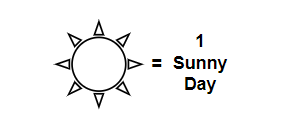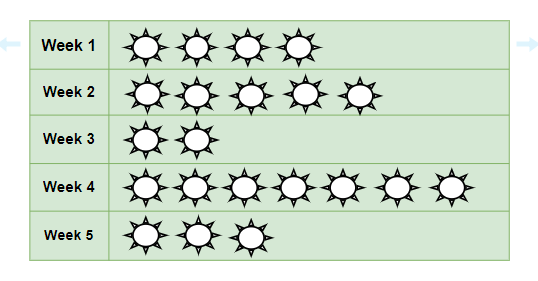### Bar Graphs

The graphical representation of any quantity, number or data in the form of bars is called a bar graph. With the help of Bar Graph, not only the data look neat and understanding but also it is easier to compare the data given.

### Types of Bar Graph

• Vertical Bar Graph
• Horizontal Bar Graph

### Vertical Bar Graph

These are the most common bar graph we come across, the bars of grouped data in vertical bar graphs lie vertically. Sometimes when the data categorized have long names, then Horizontal bar graphs are preferred since, in vertical bar graphs, there is not much space on the x-axis.

Question: There are 800 students in a school and the table for their birthdays in all 12 months is given below, Draw the Vertical Bar graph and answer,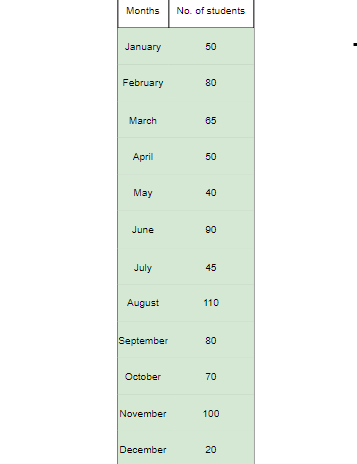1. Maximum number of students have their birthdays in which month?
2. Which two months have equal number of birthday?
3. The minimum number of birthdays occur in which month?

Solution:

The vertical bar graph for the table given in the question will be,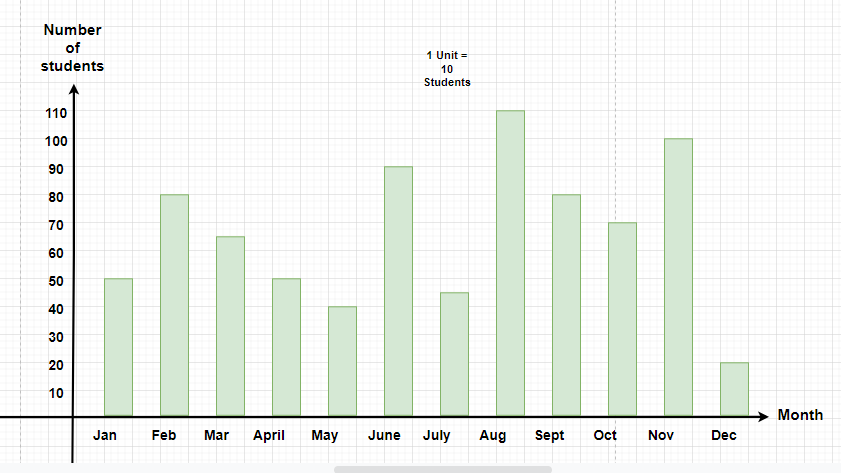From the Bar graph we can figure out the answer of the questions

1. August is that month in which maximum birthdays are happening, since the bar above august is the longest(there are 110 students whose birthday come in August)
2. From the graph, we can tell that January and April have equal lengths of bars, That means they have the same number of birthdays (both have 50 birthdays)
3. The minimum number of birthdays occur in December since it has the smallest bar.(20 students have their birthdays in December.

### Horizontal Bar Graph

The graphs that have their rectangular bars lying horizontally, which means that the frequency of the data lie on the x-axis while the categories of the data lie on the y-axis are known as Horizontal bar graphs.

Horizontal bar graphs are preferred when the name of the categories of data are long and the minimum space on the x-axis is not sufficient.

Question: In an examination, Reeta performed in 5 subjects, her performance is given in the table below. Draw a Horizontal Bar graph showing the marks she obtained in all the subjects, Also, calculate the overall Percentage obtained by her.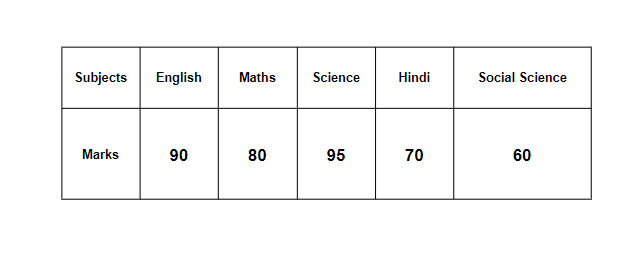Solution:

The Horizontal bar graph for the table mentioned in the question,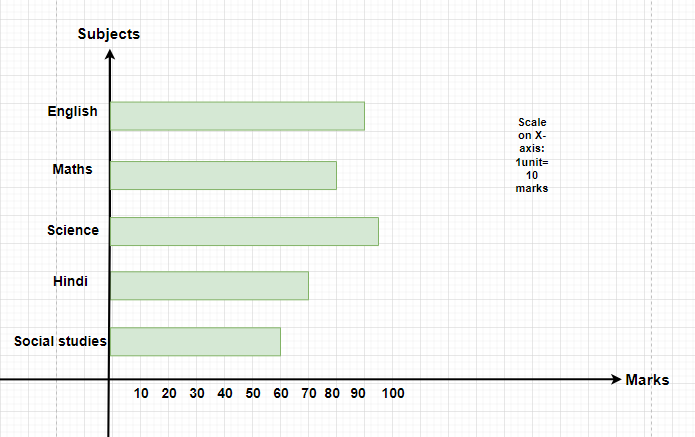The overall Percentage obtained by Reeta =×100

= 79 percent.

### Double- Bar Graph

Double-bar graphs are used when two groups of data are required to be represented on a single graph. In a double-bar graph, to represent two groups of data, they are represented beside each other at different heights depending upon their values.

Advantages of double-bar graph:

• A double-bar graph is helpful when multiple data are required to be represented.
• It helps in summarizing large and big data in an easy and visual form.
• It shows and covers all different frequency distribution.

Question 1: The table for the number of boys and girls for classes 6, 7, 8, 9, and 10 is shown below. Represent the data on a Double-bar graph.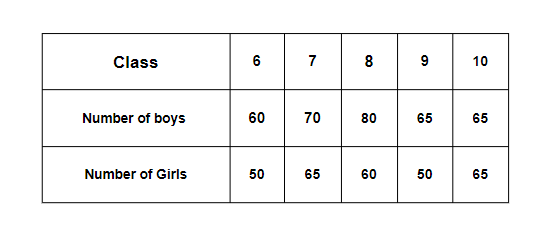Solution:

The double-bar graph for the table given the question,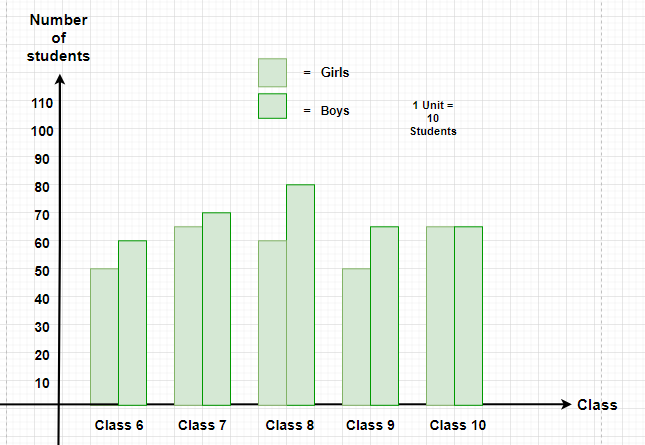Question 2: In a survey done for a week from Monday to Sunday, for two cities Agra and Delhi, The temperatures of both the cities are measured and the temperatures obtained are as following,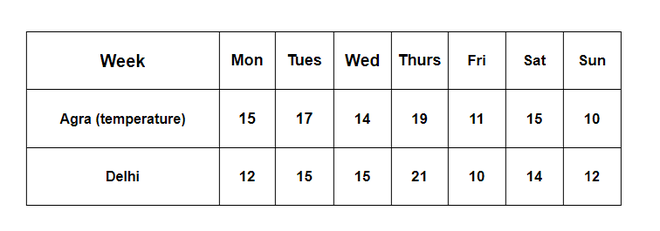Draw the Bar Graph for the given table in the question.

Solution:

The Given table has two categories of data, one is the temperature for Agra and the other is the temperature for Delhi, Therefore, the graph can be drawn in one as a double- Bar graph, the graph shall look like,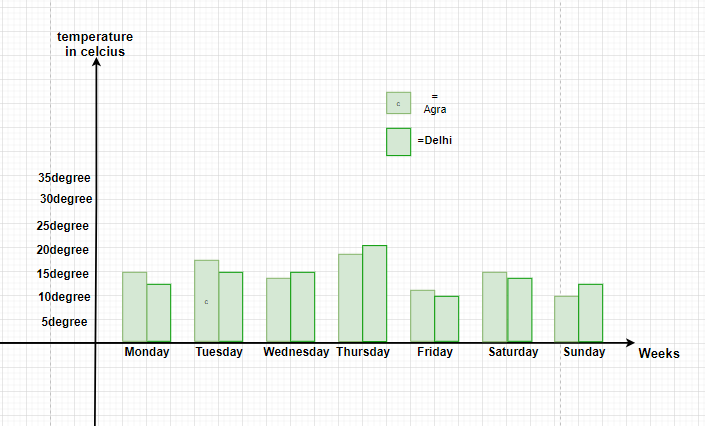My Personal Notes arrow_drop_up